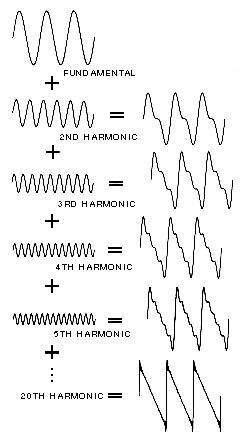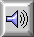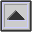LAW OF SUPERPOSITION

Two WAVEFORMs combine in a manner which simply adds their respective AMPLITUDEs LINEARally at every point in time. Thus, a complex SPECTRUM can be built by mixing together different SINE WAVEs of various amplitudes. Compare the reverse process: FOURIER ANALYSIS.

An alternative statement of the law is that two or more SOUND WAVEs may propagate in the same space simultaneously, the resultant PRESSURE variation at any point being the algebraic sum of the instantaneous pressure variations of each component wave.Successive approximations of a SAWTOOTH WAVE by addition of harmonics with amplitude inversely proportional to the harmonic number. The resultant waveform at each stage of addition is shown at right.Sound Example: Addition of the first 14 sine wave harmonics resulting in the successive approximation of a sawtooth wave.

home